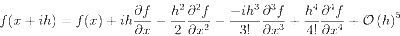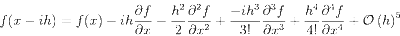## Tuesday, November 18, 2008

### Complex Step**2

I wrote a while back about using a complex step to approximate the first derivative of a function. The neat thing being that there was no subtractive cancellation in the approximation formula as there would be in a normal finite difference. This meant that we could choose very small step sizes without suffering the effects of magnified round-off error.

What about higher order derivatives? The simple result for the first derivative was a second order approximation that only required one function evaluation. Can we achieve the same benefits for a second derivative? The answer is not quite, but it's still pretty good.

The usual way to approximate a second derivative with finite differences is to take a central difference. This uses Taylor series expansions about the location of interest in two directions:Adding the idea of using complex steps gives two additional points to include in the approximation formula:Now we just have to solve a linear system to figure out what the coefficients are in our approximation formula:Based on the Taylor series expansions above, we want the real part of the answer (rather than the imaginary part as was the case with the first derivative). The coefficients are 1/2 for the functions with the real step and -1/2 for the functions with the imaginary step (and zero for the function at x). So we weren't able to avoid subtractive cancellation, but we got an explicit 4th order accurate approximation for the second derivative on a compact stencil!